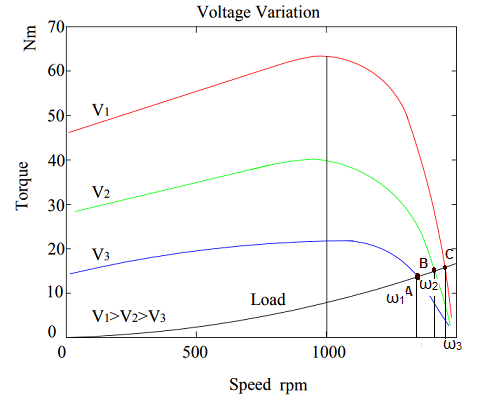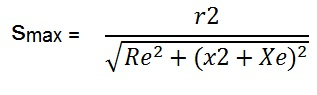# Stator Voltage Control of Induction Motor

Stator Voltage Control of Induction Motor is a method for the speed control of induction motor by changing the stator terminal voltage. Stator terminal voltage in this method is changed either by adding a series resistor, series inductor or auto transformer in stator circuit. But addition of series resistance is quite inefficient due to losses in the resistor. Therefore, modern way to have stator voltage control is to use solid state semiconductor devices like AC voltage controller or AC chopper.

Let us discuss Stator Voltage Control of Induction Motor in detail. First of all, consider the torque equation of induction motor for better understanding. As we know that, the torque is directly proportional to the square of applied stator terminal voltage, therefore

T = kV2  ……………(1)

where V = Terminal voltage of motor

k = some constant

From (1), it is clear that if we change the applied voltage, motor torque will also change. Speed torque characteristic of induction motor for three different voltages is shown below.From the above speed torque characteristic, following points can be noted:

• The slip at the maximum torque is same for all the three voltages V1, V2 and V3. This is justified as the slip Smax at maximum torque is independent of applied motor terminal voltage. It only depends on the Stator and Rotor circuit parameters as evident from its formula. The value of slip at maximum torque is given aswhere Re = Equivalent Resistance of Stator winding

x2 = reactance of rotor winding

Xe = equivalent reactance of stator winding

• The maximum torque in induction motor is directly proportional to the square of applied motor terminal voltage. Therefore, as the terminal voltage increases from V1 to V3, the maximum torque also increases. Maximum torque in induction motor is also called Stalling or Pull Out torque.
• The starting torque increases as the motor terminal voltage is increased.

Based on the above three points, we can now move on to implement these ideas on speed control of induction motor by Stator Voltage Control.

From the speed torque characteristics, we can see that, at a given motor terminal voltage, the starting torque is low and it increases as the rotor speed increases. Therefore speed control of induction motor by Stator Voltage Control, is suitable for loads requiring low starting torque but their torque requirement increases with speed. An example of this kind of load is Fan or Blower.

The variation of torque with speed for Fan / Blower load is given as

T = k ω2

where k is some constant and w is speed.

Thus the starting torque requirement is zero for such load and their torque requirement increases as speed increases. The speed torque characteristics of Fan or Blower load is shown in the above figure. It is quite clear from the above figure that:

• At terminal voltage V1, the fan will start and operate at point A with constant speed of ω1.
• The fan will operate at point B with speed ω2 for motor terminal voltage V2.
• At motor terminal voltage V3, the fan is operating with speed ω3 at point C.

Thus the load speed is controlled from ω1 to ω3 by changing the voltage from V1 to V3. This is how Stator Voltage Control of Induction Motor is used for speed control.

This site uses Akismet to reduce spam. Learn how your comment data is processed.